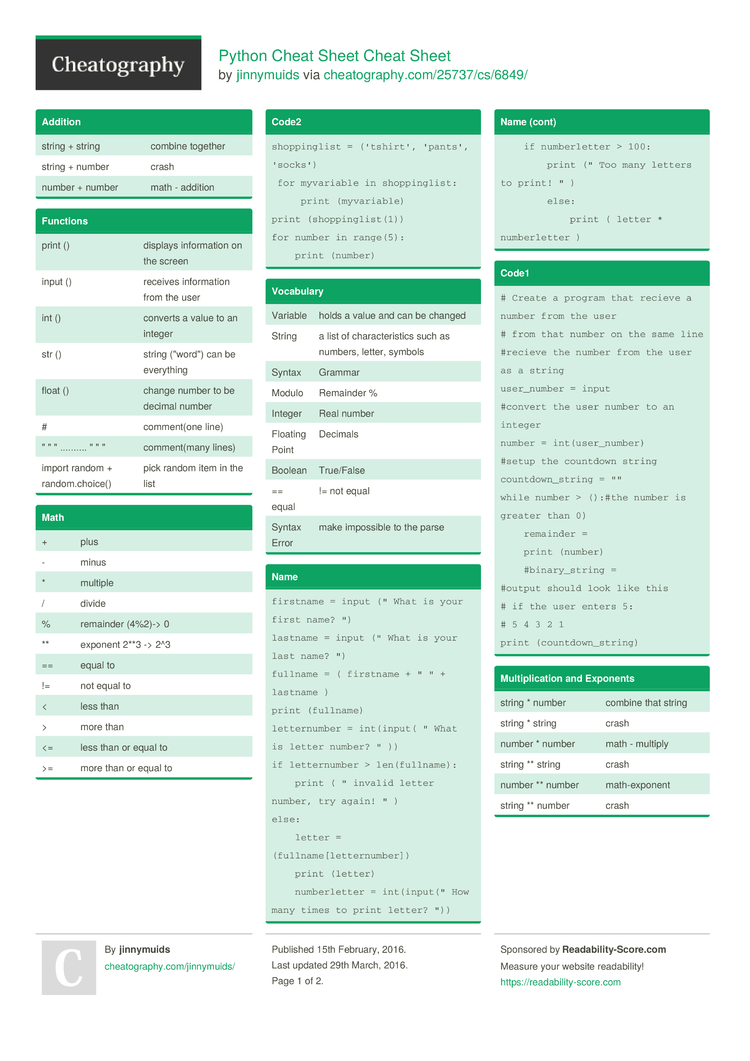Show Menu

# Python Cheat Sheet Cheat Sheet by jinnymuids

### Math

 + plus - minus * multiple / divide % remainder (4%2)-> 0 ** exponent 2**3 -> 2^3 == equal to != not equal to < less than > more than <= less than or equal to >= more than or equal to

### Addition

 string + string combine together string + number crash number + number math - addition

### Functions

 print () displays inform­ation on the screen input () receives inform­ation from the user int () converts a value to an integer str () string ("wo­rd") can be everything float () change number to be decimal number # commen­t(one line) " " " .......... " " " commen­t(many lines) import random + random.ch­oice() pick random item in the list

### Code2

 ```shoppinglist = ('tshirt', 'pants', 'socks')  for myvariable in shoppinglist:      print (myvariable) print (shoppinglist(1)) for number in range(5):     print (number)```

### Name

 ```firstname = input (" What is your first name? ") lastname = input (" What is your last name? ") fullname = ( firstname + " " + lastname ) print (fullname) letternumber = int(input( " What is letter number? " )) if letternumber > len(fullname):     print ( " invalid letter number, try again! " ) else:     letter = (fullname[letternumber])     print (letter)     numberletter = int(input(" How many times to print letter? "))     if numberletter > 100:         print (" Too many letters to print! " )         else:             print ( letter * numberletter )```

### Vocabulary

 Variable holds a value and can be changed String a list of charac­ter­istics such as numbers, letter, symbols Syntax Grammar Modulo Remainder % Integer Real number Floating Point Decimals Boolean True/False == equal != not equal Syntax Error make impossible to the parse

### Code1

 ```# Create a program that recieve a number from the user # from that number on the same line #recieve the number from the user as a string user_number = input #convert the user number to an integer number = int(user_number) #setup the countdown string countdown_string = "" while number > ():#the number is greater than 0)     remainder =     print (number)     #binary_string = #output should look like this # if the user enters 5: # 5 4 3 2 1 print (countdown_string)```

### Code

 mystring = "­hel­lo" print (mystring) firstname = input ("What is your firstn­ame­?") lastname = input ("What is your lastna­me"?) fullname = firstname + " " + lastname print(­ful­lname) letter­number = int(in­put­("What is letter number? " ) ) if lettername > len(fu­lln­ame): print ("in­valid letter number, try again! " ) else: letter = (fulln­ame­(le­tte­rnu­mber) ) print (letter) number­letter = int(input ( "How many times to print letter " ) )

### Binary

 ```user_number = input("Enter a number to convert to binary:") number = int(user_number) binary_string = '' while (number>0):        remainder = number % 2        binary_string = str(remainder) + str(binary_string)        number = number // 2 print ("Binary string is '', binary_string)```

### Area of Circle

 ```while 2==2:     #Ask the user for a radius of a circle     user_radius = input("What is the radius? ")     #Convert the given radius to a floating point     radius = float(user_radius)     #make a variable called pi     pi = 3.1415     #Calculate the area of the circle using exponents     area = pi radius * 2     #display the area of the circle to the user     print ("The area of the circle is",area)```

### Multip­lic­ation and Exponents

 string * number combine that string string * string crash number * number math - multiply string ** string crash number ** number math-e­xponent string ** number crash

## Download the Python Cheat Sheet Cheat Sheet2 Pages
//media.cheatography.com/storage/thumb/jinnymuids_python-cheat-sheet.750.jpg

PDF (recommended)

Alternative Downloads

## Comments

No comments yet. Add yours below!

## Add a Comment

Your Comment

Please enter your name.

Please enter your email address

Please enter your Comment.